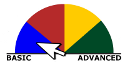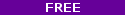# Introduction to StatisticsStatistics is about extracting meaning from data. In this class, we will introduce techniques for visualizing relationships in data and systematic techniques for understanding the relationships using mathematics. This course does not require any previous knowledge of statistics. Basic familiarity with algebra such as knowing how to compute the mean, median and mode of a set of numbers will be helpful. This course will cover visualization, probability, regression and other topics that will help you learn the basic methods of understanding data with statistics.